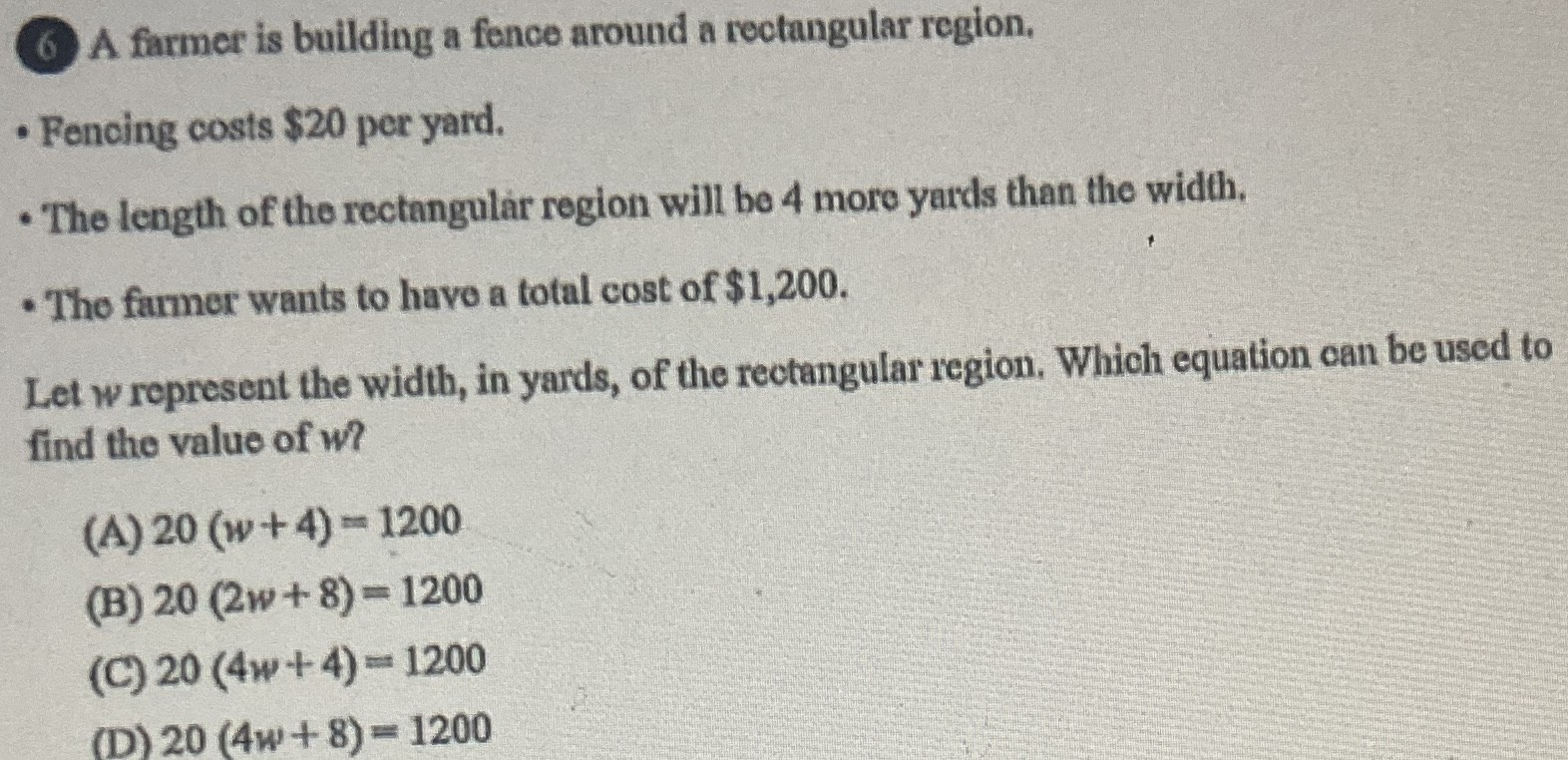### ¿Todavía tienes preguntas de matemáticas?

Pregunte a nuestros tutores expertos
Algebra
Pregunta(6) A farmer is building a fence around a reotangular region, - Fencing costs $$\ 20$$ per yard. The length of the rectangular region will be $$4$$ more yards than the width, The famer wants to havo a total cost of $$\ 1,200$$ . Let w represent the width, in yards, of the rectangular region, Which equation can be used to find the value of w?

(A) $$20 ( w + 4 ) = 1200$$

(B) $$20 ( 2 w + 8 ) = 1200$$

(C) $$20 ( 4 w + 4 ) = 1200$$

(D) $$20 ( 4 w + 8 ) = 1200$$